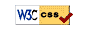# UM Math 215 On-Line Homework F23

## Hints and Tips

1. Start early, when each set opens.
2. Do the example set (homework0). It's easy & will help you with the homework system.
3. Don't submit partial answers. You have six submissions per problem.
4. Work the problems out by hand before entering them in the system. You can get a hardcopy of a problem set from the problem listing page of a problem set.
6. Use calculator syntax:
1. Carefully parenthesize. Type `cos(2pi)`, `e^(-ln(3))`, or `1/(1+x)`, not `cos 2pi`, `e^-ln3` or `1/1+x`. Note that `sin2x` is read as `sin(2)*x`, which is probably not what you want. Enter `sin(2x)` instead.
2. The square root is `sqrt(x)` or `x^(1/2)`.
7. Watch your case: `X` is different from `x`, `Sin` is different from `sin`, and `Pi` is different from `pi`. You want `pi`.

## Important InfoUM Math 215 On-Line HW